• 摩根定律
2021-04-12 16:55:09

一. 德·摩根定律

非(A且B) = (非A) 或(非B)

非(A 或B) = (非A) 且(非B)

1.德·摩根定律确实让人头疼,下面为大家具体详解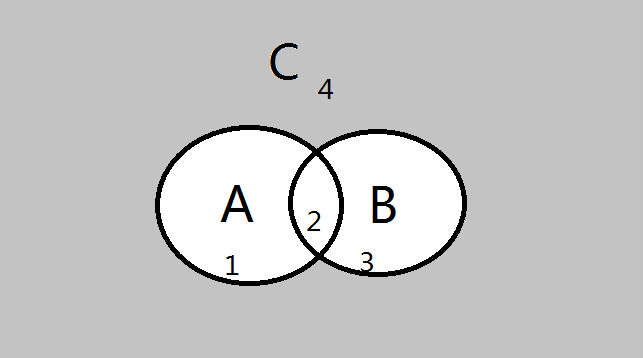由图片可知

A=1+2 ;B=2+3 ;c=4

非A=3+4

非B=1+4

A且B 是同时是A,也同时是B,所以A且B=2

A或B 既可以是A,也可以是B,所以A或B=1+2+3

所以非(A且B) =1+3+4  (非A) 或(非B) =3+4+1

得    非(A且B) = (非A)或(非B)

同理可知 非(A或B) = (非A)且(非B)

(2)在linux中组合条件为

与：-a

或：-o

非：-not, !

德·摩根定律即：

!A -a !B = !(A -o B)

!A -o !B = !(A -a B)

实例:

查找/var目录下最近一周内其内容修改过，同时属主不为root，也不是postfix的文件[root@centos7 bin]# find /var -mtime -7 -not $$-user root -o -user postfix$$

或者

[root@centos7 bin]# find /var -mtime -7 -not -user root -a -not -user postfix

二. 作业:

1、查找/var目录下属主为root，且属组为mail的所有文件[root@centos7 bin]# find /var -user root -group mail

2、查找/var目录下不属于root、lp、gdm的所有文件[root@centos7 bin]# find /var -not $$-user root -o -user lp -o -user gdm$$ -ls

3、查找/var目录下最近一周内其内容修改过，同时属主不为root，也不是postfix的文件[root@centos7 bin]# find /var -mtime -7 -not $$-user root -o -user postfix$$

4、查找当前系统上没有属主或属组，且最近一个周内曾被访问过的文件[root@centos7 bin]# find / -nouser -nogroup -atime -7 -ls

5、查找/etc目录下大于1M且类型为普通文件的所有文件[root@centos7 bin]# find /etc -size +1M -type f -ls

6、查找/etc目录下所有用户都没有写权限的文件[root@centos7 bin]# find /etc/  -not    -perm  /222 -ls

7、查找/etc目录下至少有一类用户没有执行权限的文件[root@centos7 bin]# find /etc/ -not  -perm -111 -ls

8、查找/etc/init.d目录下，所有用户都有执行权限，且其它用户有写权限的文件[root@centos7 bin]# find /etc/init.d/  -perm -113

更多相关内容
• 摩根定律的定义如下： 文字描述如下： 使用对偶性可以很方便的记忆和使用这个定律。. 我们知道如下关系呈现对偶关系，可以认为是“非”的关系： 那么将 利用对偶关系对应改写可以得到： .那么可以...

NAND = ~(a & b) = ~a | ~b

NOR = ~(a | b)  = ~a & ~b

德.摩根定律的定义如下：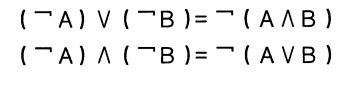文字描述如下：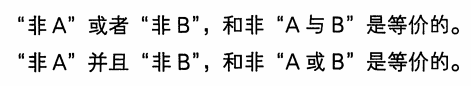使用对偶性可以很方便的记忆和使用这个定律。.

我们知道如下关系呈现对偶关系，可以认为是“非”的关系：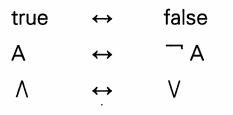那么将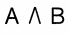利用对偶关系对应改写可以得到：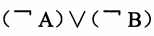.那么可以对应得到：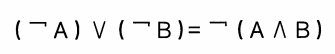通过应用德.摩根定律，有时可以简化一些逻辑问题，而使用对偶性来理解这个定律，更好的理解的同时也有利于记忆。

扩展一下：三值的德.摩根定律，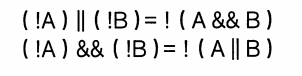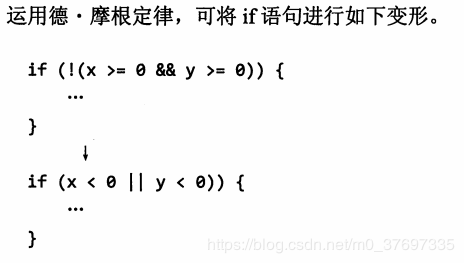vmp里面只有1个逻辑运算指令 not_not_and 设这条指令为P

P(a,b) = ~a & ~b

这条指令的神奇之处就是能模拟 not and or xor 4条常规的逻辑运算指令

怕忘记了，直接给出公式，后面的数字指需要几次P运算

not(a) = P(a,a) 1

and(a,b) = P(P(a,a),P(b,b)) 3

or(a,b) = P(P(a,b),P(a,b)) 2

xor(a,b) = P(P(P(a,a),P(b,b)),P(a,b)) 5

上面的次数应该是最少需要的次数了，当然也可以展开，那样就更加复杂了

vmp用1条指令模拟了4条指令，因此逆向起来比较复杂，如果中间夹杂垃圾运算，那么工程量非同小可

下面来证明一下上面4条等式

not(a) = ~a = ~a & ~a = P(a,a)

and(a,b) = a & b = ~(~a) & ~(~b) = P(not(a),not(b)) = P(P(a,a),P(b,b))

or(a,b) = a | b = ~(~(a|b)) = ~(~a & ~b) = ~P(a,b) = P(P(a,b),P(a,b))

xor(a,b) = ~a & b | a & ~b = ~(~(~a & b | a & ~b)) = ~(~(~a & b) & ~(a & ~b)) = ~((a | ~b) & (~a | b)) = ~(1 | 1 | a & b | ~a & ~b) = ~(a & b) & ~(~a & ~b) = P(and(a,b),P(a,b)) = P(P(P(a,a),P(b,b)),P(a,b))

上面的xor是最复杂的，不过简化后也只需要5次运算就可以实现了

至于eflag，eflag是根据结果来定的，由于都是逻辑运算，所以最后取一下eflag即可

在某修改版的vm中，还可以看到另一个强大的指令 not_not_or 设这条指令为Q

Q(a,b) = ~a | ~b

同样，这一条指令可以模拟4条常规的逻辑运算指令

怕忘记了，直接给出公式，后面数字表示需要几次Q运算

not(a) = Q(a,a) 1

and(a,b) = Q(Q(a,b),Q(a,b)) 2

or(a,b) = Q(Q(a,a),Q(b,b)) 3

xor(a,b) = Q(Q(Q(a,a),b),Q(a,Q(b,b))) 5

基本和上面P指令相同，效率没什么变化

只对最复杂的xor证明一下，以防忘记

xor(a,b) = ~a & b | a & ~b = ~(~(~a & b | a & ~b)) = ~(~(~a & b) & ~(a & ~b)) = ~((~(~a) | ~b) & (~a | ~(~b))) = ~(~(~a) | ~b) | ~(~a | ~(~b)) = Q(Q(not(a),b),Q(a,not(b))) = Q(Q(Q(a,a),b),Q(a,Q(b,b)))

实在太难了，完全搞不定啊

展开全文系统安全
• 初中数学学过集合的概念：~表示补集∩表示交集∪表示并集∈表示属于！表示取反条件:x属于C集合中之外的部分:~C证明：∵x∈~Cx属于集合c的补集x∈~(A∩B)想属于a和b集合交集的补集!(x∈A或者x∈B) (x属于A或者x属于B)...

初中数学学过集合的概念：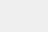~表示补集

∩表示交集

∪表示并集

∈表示属于

！表示取反

条件: x属于C集合中之外的部分:~C

证明：

∵x∈~C    x属于集合c的补集

x∈~(A∩B)    想属于a和b集合交集的补集

!(x∈A或者x∈B)    (x属于A或者x属于B)取反

x!∈A或者x!∈B    x不属于A或者x不属于B

x∈~A或者x∈~B    x属于A的补集或者x属于B的补集

x∈~A∪~B    x属于A的补集并上B的补集

∴~(A∩B)=~A∪~B

结论：A集合与B集合的交集，也就是C集合，取C集合的补集=A的补集并上B的补集

在shell中也同样通用

交集在shell中是-and

并集在shell中是-or

补集在shell中是取反！

根据上述证明得出：!(a -a b)=!a -o !b

作业：

1、查找/var目录下属主为root，且属组为mail的所有文件2、查找/var目录下不属于root、lp、gdm的所有文件3、查找/var目录下最近一周内其内容修改过，同时属主不为root，也不是postfix的文件4、查找当前系统上没有属主或属组，且最近一个周内曾被访问过的文件5、查找/etc目录下大于1M且类型为普通文件的所有文件6、查找/etc目录下所有用户都没有写权限的文件7、查找/etc目录下至少有一类用户没有执行权限的文件8、查找/etc/init.d目录下，所有用户都有执行权限，且其它用户有写权限的文件原创文章，作者：landanhero，如若转载，请注明出处：http://www.178linux.com/34588

展开全文• 今天说说德摩根定律在编程中的实践，题目看的很吓人，其实只要有一点点的高中数学知识就能看懂，而且这部分知识掌握后可以很快的运用到项目中，投资收益比非常高。如果你觉得我的文章对你有帮助，在收藏的过程中，...

今天说说德摩根定律在编程中的实践，题目看的很吓人，其实只要有一点点的高中数学知识就能看懂，而且这部分知识掌握后可以很快的运用到项目中，投资收益比非常高。

如果你觉得我的文章对你有帮助，在收藏的过程中，一定要记得点赞哦，谢谢你，这对我真的很重要🌟！

一、缘起：一段让人头大的逻辑判断

这两天在重构一些老项目，重构过程中遇到了一个让人非常头大的逻辑判断：

if(!((A && B) || C)) {

// do something

} else {

// do something

}

看了这段代码，我人都傻了，从里向外一层一层梳理逻辑时，我的大脑活动是这样的：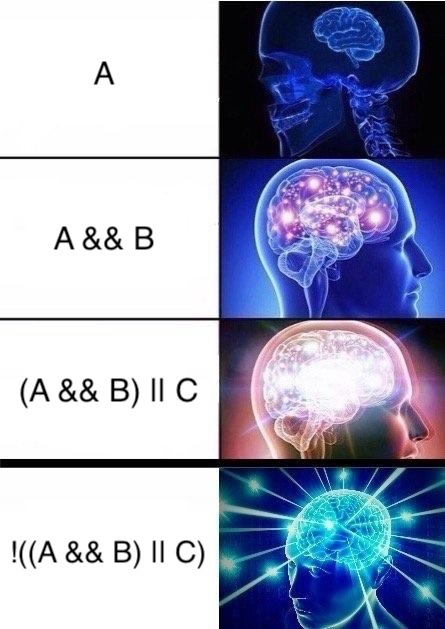短短一行的逻辑判断里，与或非三个运算符都用上了，尤其是最后那个小括号一圈全体取反的操作，我脑子直接炸了。要知道人脑是很不擅长或运算和非运算的，更不要说这些运算组合在一起了。

又花了五分钟尝试从代码上下文中梳理业务逻辑无果后，我重新审视了这个问题：如果业务上不好处理这个问题，能不能从理论上找到突破口？

方向找对后，我很快就找到了解决方案，那就是离散数学里的德摩根定律(De Morgan’s laws)。

二、什么是德摩根定律

德摩根定律我们其实很早就接触过了，高中数学的集合部分就讲过，大学离散数学的集合运算和布尔代数部分也有所提及。

德摩根定律在离散数学的很多场景里都出现过，它一共有两个关系：

在命题逻辑里，可以这样表示：

$$\neg (P\lor Q)\iff (\neg P)\land (\neg Q)$$

$$\neg (P\land Q)\iff (\neg P)\lor (\neg Q)$$

其中 $\neg$ 表示逻辑非运算符(NOT, !)，$\lor$ 表示逻辑或运算符(OR, ||)，$\land$ 表示逻辑与运算符(AND, &&)

在集合里可以这样表示：

$$\overline {A\cup B} = \overline {A} \cap \overline {B}$$

$$\overline {A\cap B} = \overline {A} \cup \overline {B}$$

其中 A 和 B 表示集合，集合上的横线表示取补集，$\cap$ 表示取交集，$\cup$ 表示取并集。

在布尔代数里可以这样表示：

$$\overline {(x \cdot y)} = \overline {x} + \overline {y}$$

$$\overline {(x+y)} = \overline {x} \cdot \overline {y}$$

其中 $\cdot$ 表示布尔积(AND)，$+$ 表示布尔和(OR)，上划线表示补(NOT)。

上面的公式还可以用文字描述：

非($P$ 或 $Q$)等价于(非 $P$)且(非 $Q$)

非($P$ 且 $Q$)等价于(非 $P$)或(非 $Q$)

或者用程序员熟悉的与或非逻辑运算符表示：

!(P || Q) = !P && !Q

!(P && Q) = !P || !Q

公式已经摆在这里了，肯定可以直接用了，但是用的时候还是不太放心，最好还是自己验证一下。我们可以用真值表对 $\neg (P\land Q)\iff (\neg P)\lor (\neg Q)$ 做个直观的验证：

$P$

$Q$

$\neg P$

$\neg Q$

$(P\land Q)$

$\neg (P\land Q)$

$(\neg P)\lor (\neg Q)$

0

0

1

1

0

1

1

0

1

1

0

0

1

1

1

0

0

1

0

1

1

1

1

0

0

1

0

0

我们也可以用 Venn 图对 $\overline {A\cap B} = \overline {A} \cup \overline {B}$ 做个可视化验证：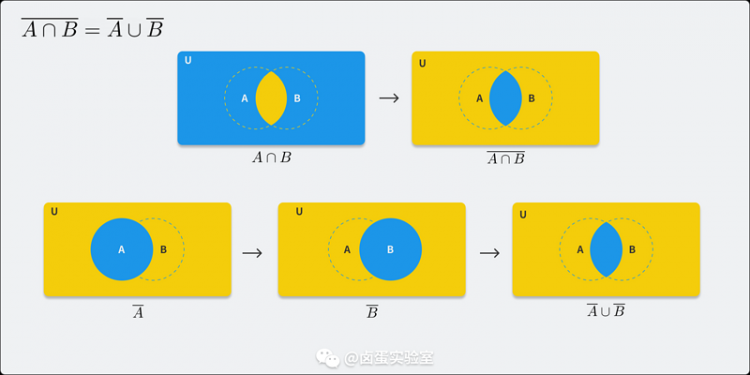更复杂的数学推导我这里就不多说了，感兴趣的同学可以自行搜索学习。

三、解决问题

具体到我一开始说的那个条件判断上，我们可以用德摩根定律把原表达式拆开：

!((A && B) || C)

== !(A && B) && !C // 德摩根律

== (!A || !B) && !C // 德摩根律

== (!A && !C) || (!B && !C) // 分配律

用分配律转化后，经过代码的上下文分析，我发现在这段代码的业务场景中， !A 等价于 C，所以上面的式子还可以化简：

(!A && !C) || (!B && !C)

== (C && !C) || (!B && !C) // !A 等价于 C(从业务上分析的，不具备普遍意义)

== false || (!B && !C) // C && !C 必定为 false

== !B && !C

== A && !B // A 等价于 !C(从业务上分析的)

到这里，我成功的把原来一段让人脑袋爆炸的判断语句化简为一段直白易懂的表达式，转换后的代码无论是从理解上还是后期维护上都比原来容易很多。

四、化简还有什么招？

与或非三者一起用的场景可以尝试用德摩根定律化简，在其它场景下，还可以利用其它运算律进行转换，比如说前面我就用了分配律。类似于积之和展开式的化简，我们还可以用卡诺图进行分析。

例如一个场景试图化简布尔函数的一个积之展开式：$x\overline {y} + \overline {x}y + \overline {x} \overline {y}$ ，就可以用卡诺图进行分析：

$y$

$\overline {y}$

$x$

1

$\overline {x}$

1

1

根据图示可以轻易得出最后的化简结果为 $\overline {x} + \overline {y}$。

如果变量超过 4 个，卡诺图就非常复杂了，这时候可以用奎因-莫克拉斯基方法进行化简，限于篇幅也不多介绍了，感兴趣的同学可以自行搜索。

在此我再多说一句，如果真的出现用 4 个以上的子状态去决定最终的行为时，问题的关键就不是化简，而是顶层设计上就出了问题了。这时候需要停下来好好思考是不是模型的设计抽象程度不够，把过多的内部状态暴露了出来等等，这个得具体业务具体分析，一昧的堆 if else 或加 flag 是解决不了问题的，只会导致代码的腐化最终无法维护。

最后推荐一波我的微信公众号：卤蛋实验室和我的个人博客：supercodepower.com, 目前专注前端技术，对图形学也有一些微小研究，欢迎大家来撩～

五、推荐阅读

【答疑解惑】为什么你的 Charles 会抓包失败？

webpack 中那些最易混淆的 5 个知识点

React Native 性能优化指南【全网最全，值得收藏】

展开全文• 证明德摩根定律 X / (A U B) = (X \ A) 交 (X \ B) 和 X \ (A 交 B) = (X \ A) U (X \ B) 不妨先设几个数据一下看看定律是否正确: A = {1, 2, 3, 4} B = {2, 4, 6} X = {1, 2, 3, 4, 5, 6} 易得 A U B 为 {1, 2, 3...
• ## 德-摩根定律

万次阅读 2017-07-29 02:06:52
德·摩根定律在数理逻辑的定理推演中，在计算机的逻辑设计中以及数学的集合运算中都起着重要的作用。 他的发现影响了乔治·布尔从事的逻辑问题代数解法的研究。这巩固了德摩根作为该规律的发现者的地位，尽管...数学 设计 class
• 摩根定律的定义如下： 文字描述如下： 使用对偶性可以很方便的记忆和使用这个定律。. 我们知道如下关系呈现对偶关系，可以认为是“非”的关系： 那么将 利用对偶关系对应改写可以得到： .那么可以...
• 集合关系证明的基本原则 外延性原则 证明两个集合互相包含 当有基本结论时，就可以直接用而不需要全用外延性原则。 对S−>->−>P or Q形式的证明思路 ...德摩根定律 交对减的分配 ...
• 带那个变量A和常量0，和1之间的运算规则 ...布尔代数中的最重要的两个定律—反演律，也叫做摩根定理 可以用真值表验证其正确性 这种完全归纳法可以证明先前的所有定理 其他常用公式 吸收律 证明 ...
• 摩根定律 证明 看了一些文章，发现一个比较好理解德摩根定律的方法： 假设有 n 件事（A1，A2， A3… An）。 不做 A1 或不做 A2 或不做 A3 或 …或不做 An ——等价于——不全做 数学符号表达即为 不做 A1 也不做...数学
• 高中数学第一章常用逻辑用语1.3简单的逻辑联结词德摩根定律素材新人教A版选修2_1
• De Morgan’s laws 1. 德摩根定律与 σ 代数
• ## 证明德摩根定律

千次阅读 2018-03-31 15:06:00
1 #include<iostream> 2 using namespace std; 3 4 int main(){ 5 unsigned int a ,b; 6 for(a=0;a<256;a++){ 7 for(b=0;b<256;b++){ 8 if((~(a&am...
• 1.德摩根定律（1）初悉德摩根定律儿在命题逻辑和逻辑代数中，德·摩根定律（或称德·摩根定理）是关于命题逻辑规律的一对法则。 奥古斯塔斯·德·摩根首先发现了在命题逻辑中存在着下面这些关系： 非(A且B)=(非A)或...
• Linux中的德·摩根定律§·德·摩根定律介绍※概念在命题逻辑和逻辑代数中，德·摩根定律(或称德·摩根定理)是关于命题逻辑规律的一对法则。奥古斯塔斯·德·摩根首先发现了在命题逻辑中存在着下面这些关系：非(P且Q...
• 德·摩根定律让学习liunx的小伙伴绕得头疼，现在我就用洪荒之力为大家理顺一下这个定律。由图片可以看出，A=4+3B=3+2非A=2+1非B=4+1A且B就是代表既是A又是B的地方，A和B都有色块3，所以色块3代表了A且B同时，我们再...
• 今天说说德摩根定律在编程中的实践，题目看的很吓人，其实只要有一点点的高中数学知识就能看懂，而且这部分知识掌握后可以很快的运用到项目中，投资收益比非常高。如果你觉得我的文章对你有帮助，在收藏的过程中，...
• //78 program test; var j,k:byte; begin randomize; //有这句，每次取得的随机数会不同。 j:=random(256); //取得256以内的随机数 k:=random(256); writeln( j,' ',k ); writeln;... w...数学基础
• 集合论 实变函数中集合论的刻画 集合：抽象的集合，在抽象代数中研究其中的运算，而在实变函数中注重研究集合与集合之间的关系 如何描述一个集合中元素的多少：用映射的概念引入两个集合之间元素的多少。...
• ## 集合(德摩根证明)

万次阅读 2018-06-21 10:25:29
摩根定律 ( ⋃ n S n ) c = ⋂ n S c n ( ⋃ n S n ) c = ⋂ n S n c \bigg(\bigcup_n S_n\bigg)^c = \bigcap_n S_n^{c} 证明：设   x ∈ ( ∪ n S n ) c   x ∈ ( ∪ n S n ) c \ x \in (\cup_n S_n)^c ......